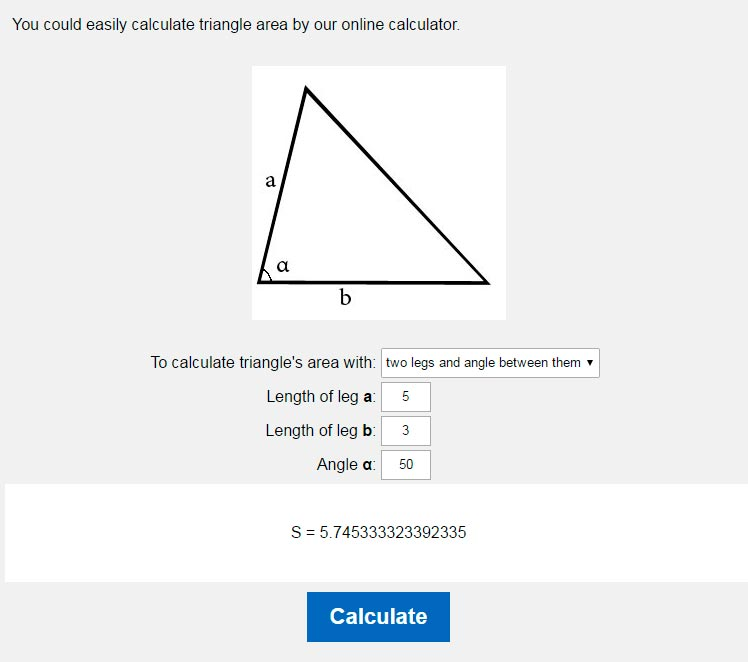# Area of a triangle calculator.

You could easily calculate triangle area by our online calculator.To calculate triangle's area with: base and heighttwo legs and angle between themGeron's formula Triangle's height h: Length of leg b:

There are several formulas by which you can calculate triangle's area.

1. If we know triangle's height and base we could calculate it's area by the next formula:
S=1/2bh2. Also you could calculate triangle's area by using Geron's formula, by which it can be done if you know perimeter or length of all it's legs:
S= √p(p-a)(p-b)(p-c)
where p - half-perimeter of triangle3. As well as in examples above you may calculate triangle's area by two legs' length and angle between them:
S=1/2ab sin(α)### The area of the triangle through the base and height### The area of the triangle through Heron's formula through knowing the perimeter and the length of all sides### The area of the triangle and two sides of the angle between themHOME
RESOURCES